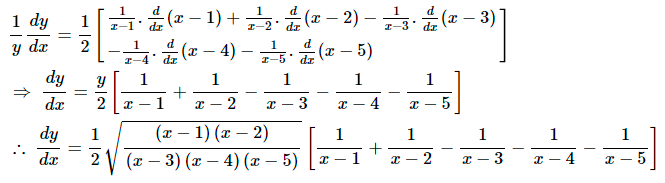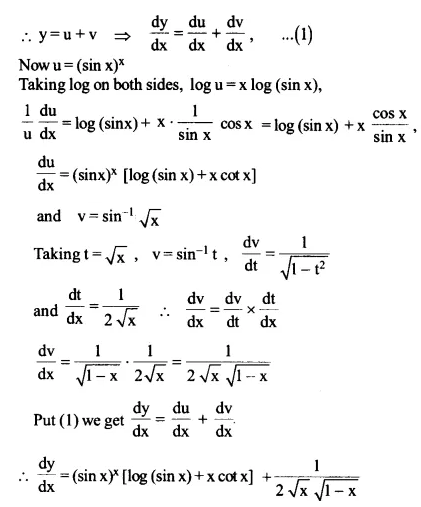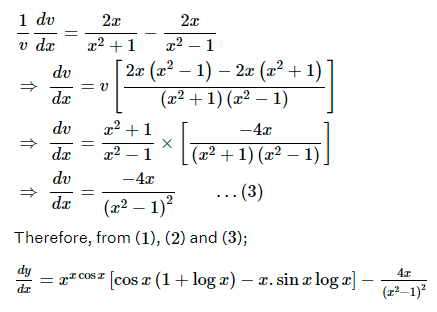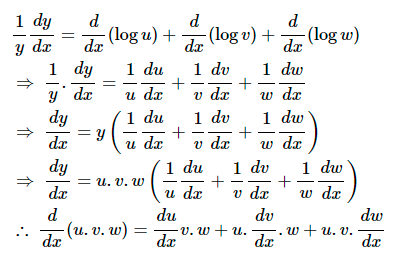NCERT Solutions for Maths Class 12 Exercise 5.5

# NCERT Solutions for Maths Class 12 Exercise 5.5

## NCERT Solutions for Maths Class 12 Exercise 5.5

Differentiate the functions given in Questions 1 to 11 w.r.t. x.

Maths Class 12 Ex 5.5 Question 1.
cos x. cos 2x. cos 3x

Solution:
Let y = cos x. cos 2x . cos 3x
Taking log on both sides, we get
log y = log (cos x. cos 2x. cos 3x)
log y = log cos x + log cos 2x + log cos 3x
Differentiating w.r.t. x, we get

Differentiating both sides w.r.t. x, we getMaths Class 12 Ex 5.5 Question 3.
(log x)cos x

Solution:
Let y = (log x)cos x
Taking log on both sides, we get
log y = log (log x)cos x
log y = cos x log (log x)
Differentiating both sides w.r.t. x, we getMaths Class 12 Ex 5.5 Question 4.
xx – 2sin x

Solution:
Let y = xx – 2sin x
y = u – v, where u = xx and v = 2sin x

Maths Class 12 Ex 5.5 Question 5.
(x + 3)2 . (x + 4)3 . (x + 5)4

Solution:
Let y = (x + 3)2 . (x + 4)3 . (x + 5)4
Taking log on both side, we get
log y = log [(x + 3)2 . (x + 4)3 . (x + 5)4]
= log (x + 3)2 + log (x + 4)3 + log (x + 5)4
log y = 2 log (x + 3) + 3 log (x + 4) + 4 log (x + 5)
Differentiating w.r.t. x, we get

Differentiating both sides w.r.t. x, we get

Taking log on both sides, we getDifferentiating both sides w.r.t. x, we getMaths Class 12 Ex 5.5 Question 7.
(log x)x + xlog x

Solution:
Let y = (log x)x + xlog x = u + v
Where u = (log x)x
Taking log on both sides, we get

log u = x log(log x)

Differentiating both sides w.r.t. x, we get

Maths Class 12 Ex 5.5 Question 8.

(sin x)x + sin-1 √x

Solution:
Let y = (sin x)+ sin-1 √x
Let u = (sin x)x and v = sin-1 √xMaths Class 12 Ex 5.5 Question 9.
xsin x + (sin x)cos x

Solution:
Let y = xsin x + (sin x)cos x = u + v
Where u = xsin x
log u = sin x log xTaking log on both sides, we get

log u = log (xxcos x)

log u = x cos x log x

Differentiating both sides w.r.t. x, we get

Taking log on both sides, we get

log v = log (x2 + 1) – log (x2 − 1)

Differentiating both sides w.r.t. x, we getSolution:

Putting the values from equations (ii) and (iii) in equation (i), we getFind dy/dx of the functions given in Questions 12 to 15.

Maths Class 12 Ex 5.5 Question 12.
xy + yx = 1

Solution:
We have, xy + yx = 1
Let u = xy and v = yx
u + v = 1,Now, u = xy

Taking log on both sides, we get

log u = log xy

log u = y log x

Differentiating both sides w.r.t. x, we get

Now, v = yx

Taking log on both sides, we get

log v = log yx

log v = x log y

Differentiating both sides w.r.t. x, we getMaths Class 12 Ex 5.5 Question 13.
y= xy

Solution:
We have, y= xy
Taking log on both sides, we get
x log y = y log x

Differentiating both sides w.r.t. x, we get

Maths Class 12 Ex 5.5 Question 14.

(cos x)y = (cos y)x

Solution:
We have, (cos x)y = (cos y)x
Taking log on both sides, we get

y log (cos x) = x log (cos y)

Differentiating both sides w.r.t. x, we get

Maths Class 12 Ex 5.5 Question 15.

xy = e(x - y)

Solution:
We have, xy = e(x - y)
Taking log on both sides, we get

log (xy) = log e(x - y)
log (xy) = (x – y) log e
log x + log y = x – y                        (Since log e = 1)

Differentiating both sides w.r.t. x, we get

Maths Class 12 Ex 5.5 Question 16.

Find the derivative of the function given by f(x) = (1 + x) (1 + x2) (1 + x4) (1 + x8) and hence find f'(1).

Solution:
Let f(x) = y = (1 + x) (1 + x2) (1 + x4) (1 + x8)
Taking log both sides, we get
log y = log [(1 + x) (1 + x2) (1 + x4) (1 + x8)]
log y = log(1 + x) + log (1 + x2) + log(1 + x4) + log(1 + x8)Maths Class 12 Ex 5.5 Question 17.

Differentiate (x2 – 5x + 8) (x3 + 7x + 9) in three ways mentioned below:
(i) by using product rule
(ii) by expanding the product to obtain a single polynomial.
(iii) by logarithmic differentiation.
Do they all give the same answer?

Solution:
(i) By using product rule,

Let y = (x2 – 5x + 8) (x3 + 7x + 9)

Let u = (x2 – 5x + 8) and v = (x3 + 7x + 9)

(ii) By expanding the product to obtain a single polynomial, we get

y = (x− 58(x79)

= x2(x79− 5(x798(x79)

= x7x9x− 5x− 35x− 458x5672

y = x− 5x15x− 26x1172
Differentiating both sides w.r.t. x, we get

(iii) By logarithmic differentiation

y = (x2 − 5x + 8) (x3 + 7x + 9)

Taking log on both the sides, we get

log y = log (x2 − 5x + 8) + log (x3 + 7x + 9)

Differentiating both sides with respect to x, we get

From the above three observations, it can be seen that all the results of dy/dx

Maths Class 12 Ex 5.5 Question 18.
If u, v and w are functions of x, then show thatin two ways - first by repeated application of product rule, second by logarithmic differentiation.

Solution:
Let y = u.v.w
y = u. (v.w)
By applying product rule, we get

Taking logarithm on both the sides of the equation y = u.v.w, we get

log y = log u + log v + log w

Differentiating both sides w.r.t. x, we get